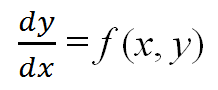## How should mathematical theories be cited in my prose and in my works-cited list?

It depends on whether the theory can be considered common knowledge.

When you discuss a complex mathematical theory, you should cite a source that explains the theory. As always, to create your works-cited-list entry for the source, follow the MLA format template and key your in-text citation to the first element of the entry. In the following example, the authors of the source, Earl D. Rainville and Phillip E. Bedient, are named in the prose, and this information directs your reader to their work in the works-cited list:

Earl D. Rainville and Phillip E. Bedient explain a “theorem concerning the existence and uniqueness of solutions” as follows:

In rough language, the theorem states that if f (x, y) is sufficiently well behaved near the point (x0, y0) then the differential equationhas a solution that passes through the point (x0, y0) and that solution is unique near (x0, y).  (19)

#### Work Cited

Rainville, Earl D., and Phillip E. Bedient. Elementary Differential Equations. 6th ed., Macmillan Publishing, 1981.

If, however, you are discussing a well-known theorem such as the Pythagorean theorem (a2 + b2 = c2), a citation may generally be omitted.